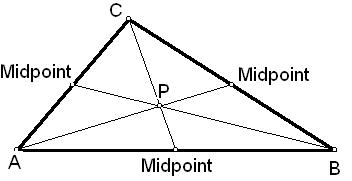# Calculate Median of a Triangle - Analytical Calculator

Calculate the median of a triangle from the given points of A, B and C.

Please enter the coordinates of a triangle
 Points X Y A B C

The triangle has exactly three medians from three vertexes which are intersecting each other at the triangle's centroid. The median of a triangle is a line segment joining a vertex to the midpoint of the opposing side. The three medians divide the triangle into 6 smaller triangles having the same area irrespective of different shapes.

### Formula:

ma = (1/2) √2c2 + 2b2 - a2

mb = (1/2) √2c2 + 2a2 - b2

mc = (1/2) √2a2 + 2b2 - c2

Where

a,b,c - Length of triangle sides.### Properties of Median

The median cannot be manipulated algebraically. The median value is fixed by its position and is not reflected by the individual value. The distance between the median and the rest of the values is less than the distance from any other point. Every array has a single median.

### The properties of the median of a triangle

In geometry, a median of a triangle is a line segment joining a vertex to the midpoint of the opposite side, thus bisecting that side, every triangle has exactly three medians, one from each vertex, and they all intersect each other at the triangle's centroid as shown in above picture